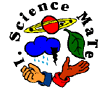Applied Science THIRD GRADE 8 week unit 1. Students skills on predicting should be increased. 2. Students should be able to contrast and compare objects geometrically. 3. Students should know basic measuring equipment. 4. Students should be able to measure volume. 5. Students should know the difference and similarities of inventors and scientists. 6. Students should understand how discoveries are made. 7. Students should understand that the movement of electrons are important in the study of electricity and magnetism. 8. Students should recognize that electricity is the flow of electrons. 9. Students should understand that magnetism is electrons that spin in the same direction. 10. Students should understand the importance of electricity in society. 11. Students should be able to evaluate electrical safety. 12. Students should recognize watt, volt, and ampere. 13. Students should recognize transportation systems to and from a city. 14. Students should understand the importance of the train to American history.  OVERVIEW OF THIRD GRADE SCIENCE AND MATH WEEK 1. PRE: Comparing objects mathematically. LAB: Predicting and measuring objects. POST: Comparing and contrasting objects. WEEK 2. PRE: Discovering lab equipment. LAB: Measuring volume using a graduated cylinder. POST: Comparing volume, mass, and weight. WEEK 3. PRE: Investigating a "new" discovery. LAB: Experimenting with peanuts. POST: Comparing inventors and scientists. PHYSICS WEEK 4. PRE: Exploring magnetism. LAB: Discovering magnetic force. POST: Comparing objects that are magnetic and non-magnetic. WEEK 5. PRE: Comparing static and current electricity. LAB: Exploring the origin of static electricity. POST: Investigating lightning. TECHNOLOGY WEEK 6. PRE: Exploring DC and AC current. LAB: Comparing series and parallel circuits. POST: Discovering how machines operate using electricity. WEEK 7. PRE: Investigating how you pay your energy bill. LAB: Exploring small appliances. POST: Evaluating electrical safety. BUILT ENVIRONMENT WEEK 8. PRE: Comparing different modes of transportation. LAB: Designing a train route to service a community. POST: Investigating different types of trains.

[Back to Applied Science Grid]